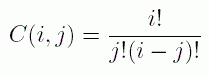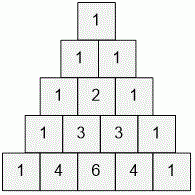Competitions

Combination

How many numbers are divisible by the prime number p in the first n rows of Pascal Triangle? In other words, find the number of pairs (j, i) (0ji < n) so that C(i, j) is divisible by p. HereInput

The first line contains two integer numbers n, p (1n107, 3p100).